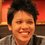# Prove (or disprove): If $\lceil x\lfloor x\rfloor\rceil+\lfloor x\lceil x\rceil\rfloor=A$ has real solutions of $x$ with positive range of $\alpha\le x\le\beta$, then $A=\alpha\lceil\ \sqrt{A/2}\ \rceil+\beta\lfloor\ \sqrt{A/2}\ \rfloor$.

Like the title said:

Is it true that if $\lceil x\lfloor x\rfloor\rceil+\lfloor x\lceil x\rceil\rfloor=A$ has real solutions of $x$ with positive range of $\alpha\le x\le\beta$, then $A=\alpha\left\lceil\ \sqrt{\frac A2}\ \right\rceil+\beta\left\lfloor\ \sqrt{\frac A2}\ \right\rfloor$ must be true?

Inspired by Raghav Vaidyanathan which was inspired by Pi Han Goh.Note by Pi Han Goh
6 years, 2 months ago

This discussion board is a place to discuss our Daily Challenges and the math and science related to those challenges. Explanations are more than just a solution — they should explain the steps and thinking strategies that you used to obtain the solution. Comments should further the discussion of math and science.

When posting on Brilliant:

• Use the emojis to react to an explanation, whether you're congratulating a job well done , or just really confused .
• Ask specific questions about the challenge or the steps in somebody's explanation. Well-posed questions can add a lot to the discussion, but posting "I don't understand!" doesn't help anyone.
• Try to contribute something new to the discussion, whether it is an extension, generalization or other idea related to the challenge.

MarkdownAppears as
*italics* or _italics_ italics
**bold** or __bold__ bold
- bulleted- list
• bulleted
• list
1. numbered2. list
1. numbered
2. list
Note: you must add a full line of space before and after lists for them to show up correctly
paragraph 1paragraph 2

paragraph 1

paragraph 2

[example link](https://brilliant.org)example link
> This is a quote
This is a quote
    # I indented these lines
# 4 spaces, and now they show
# up as a code block.

print "hello world"
# I indented these lines
# 4 spaces, and now they show
# up as a code block.

print "hello world"
MathAppears as
Remember to wrap math in $$ ... $$ or $ ... $ to ensure proper formatting.
2 \times 3 $2 \times 3$
2^{34} $2^{34}$
a_{i-1} $a_{i-1}$
\frac{2}{3} $\frac{2}{3}$
\sqrt{2} $\sqrt{2}$
\sum_{i=1}^3 $\sum_{i=1}^3$
\sin \theta $\sin \theta$
\boxed{123} $\boxed{123}$

Sort by:

Hmm , nice inspirations !

- 6 years, 2 months ago

  1 2 3 4 5 6 7 8 9 10 11 12 13 14 15 16 17 18 19 20 21 22 23 24 25 26 27 28 29 30 31 32 33 34 35 36 37 #include #include #include #include #include double fccf(double x) { double d=ceil(x*floor(x))+floor(x*ceil(x)); return d; } int main() { clrscr(); double x,l,g={0,0},n; int r=0; cin>>n; l=floor(sqrt(n/2));l=ceil(sqrt(n/2)); for(x=l;x<=l;x+=0.0001) { double k=fccf(x); if(k==n&&r==0) { g=x; r=1; } else if(k>n&&r==1) { g=x; break; } } cout<

C++ code... Tested for a lot of numbers.. seems that it isn't always true... especially in the vicinity of numbers of form $2n^2$

- 6 years, 2 months ago

So you've found a counterexample? Can you show me which values of $A$ shows that is not true?

- 6 years, 2 months ago

I think $A=129$ doesn't satisfy. And also, numbers like $A=128$ have $\alpha=\beta$.

- 6 years, 2 months ago

There's no solution for $x$ when $A=129$ so it doesn't satisfy the condition. Well technically, if $A$ is in the form of $2B^2$ for some integer $B$, then $\alpha = \beta$, so by squeeze theorem, $x = \alpha = \beta$ which doesn't contradict the statement. In other words, it's trivial to pointless to disprove this statement when $x$ is an integer.

- 6 years, 2 months ago

I agree with you on numbers of the form $2n^2$ and also numbers which don't yield a solution. Now try $A=137$...

- 6 years, 2 months ago

Because $8 < x < \frac{73}9$ is the range for $x$ when $x=137$, by applying the inequality, we can have $\alpha =8 + \epsilon, \beta = \frac{73}{9} - \epsilon$ for an extremely small positive value $\epsilon$. In other words $\alpha \gtrsim 8, \beta \lesssim \frac{73}9$.

So my statement still holds.

If this is a cop out explanation, then you've successfully disproven my statement! (and I can't have that, haha!)

- 6 years, 2 months ago

The values of $\alpha, \beta$ are one thing, but you may notice that: $9\alpha+8\beta \ne 137$. Counterexample.

- 6 years, 2 months ago

Oh I failed my math. D=

Okay this is disproven! Thank you for your cooperation. I knew this is too good to be true.

- 6 years, 2 months ago

Follow up (or modified) question: Is it true that if $\lceil x\lfloor x\rfloor\rceil+\lfloor x\lceil x\rceil\rfloor=A$ has real solutions of $x$ with positive range in a closed interval $[\alpha, \beta ]$ for $\alpha \ne \beta$, then $A=\alpha\left\lceil\ \sqrt{\frac A2}\ \right\rceil+\beta\left\lfloor\ \sqrt{\frac A2}\ \right\rfloor$ must be true?

That means to say that we can't take $A=137$ as an example because the range of solution of positive $x$ is an open interval: $\left(8, \frac{73}9\right)$.

- 6 years, 2 months ago

@Pi Han Goh Sir , does the equality hold on the upper limit side?

- 3 years ago

I don't understand your question at all. The claim clearly states that we have to first find $\alpha$ and $\beta$.

- 3 years ago